|

# 夏季装修7大好处!

炎炎夏日，这样的高温对于一大半户外的工作者来说，简直是煎熬。在这样的天气下坚持给你家装修的师傅们，真心要给他们鼓个掌！别的不多说，多给他们备点防晒工具和饮用水吧！而且夏季装修也

是检验材料的最好时机！下面来看看夏季装修的7大好处和注意事项：

好处一：容易辨别材料是否环保

夏季装修由于气温较高，装修材料中的一些有害物质加速挥发，有些材料用鼻子就能大致推断是否环保了，所以夏季装修更利于新房装修的环保性哦！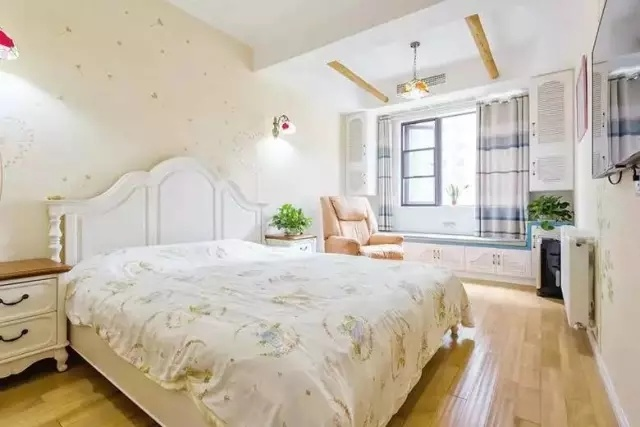好处二：夏季装修油漆效果好

大家都知道温度高气体挥发快，所以夏季装修油漆干得快，打磨也更及时，油漆的亮光度能充分体现，刷出的漆面效果佳；夏季虽然雨也多，但毕竟不会连续几天的大暴雨，对装修的影响并不大。好处三：瓷砖粘贴更牢固

夏季天气热水分蒸发过快，装修工人为了保证瓷砖充分吸水，多数会提前浸泡，有的还要进行二次浸水，这样吸饱水分的瓷砖能够很好的粘在地面和墙上。瓷砖贴完之后再用水浇一遍，打开窗户通风自然晾干，瓷砖也能粘得更为牢固。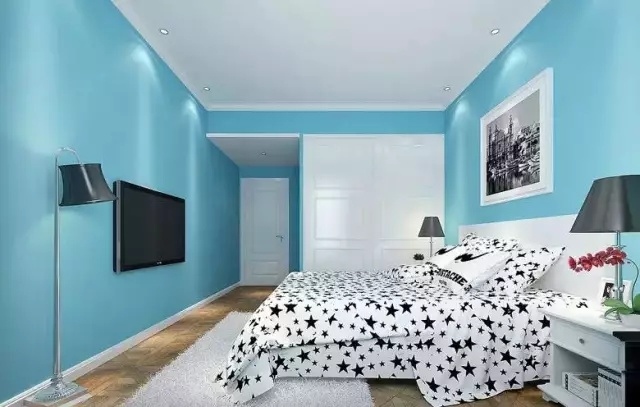好处四：便于杜绝墙面问题

夏季装修墙面处理的效果更好，刷漆前刮的腻子能够慢慢干透，而不是“表干”，这样就不会造成刷漆后涂料脱落，影响墙面的施工效果；这也就从根本上避免了常见的墙体粉化、起泡和开裂问题。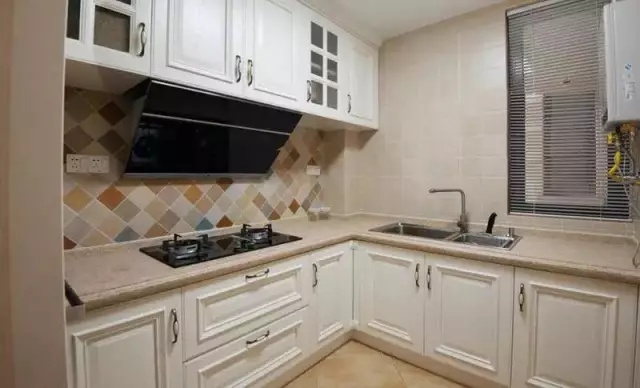好处五：夏季施工效果好

夏天装修较好还有一个原因就是完工之后如果真的有质量不好的问题，也能够较早的反映出来以便修补；到了秋天空气干燥，如果真有木地板之间缝隙加大、墙纸开裂等问题都容易发现尽早处理，而这也都在装修保修期更利于及时修补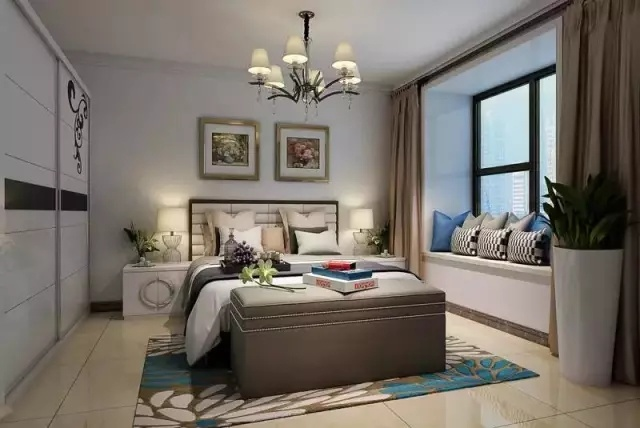好处六：夏季装修工期短

夏季是传统装修淡季，所以装修公司装修生意少，装修工人不用同时干几家的装修活，也不像冬季要赶着回家过年，所以不会因为赶工而偷工减料，房子装修质量有保证。

好处七：获得意想不到的好处

虽说夏季是传统的装修淡季，但越来越多的装饰公司利用“淡季”做文章，让利促销活动不断，业主还可以抓住机遇得到不少实惠，而且在传统的淡季手艺好的工人也更容易挑选得到。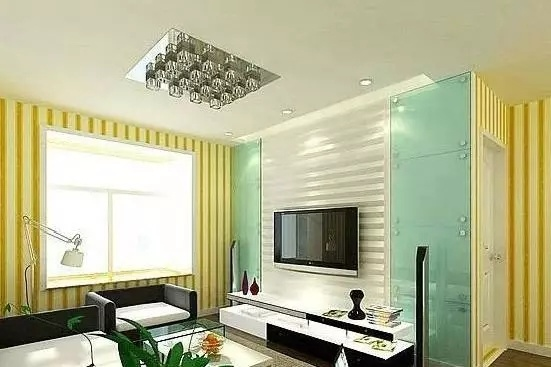夏季装修注意事项

施工注意防潮

夏季是一个雨水多的季节，而且夏季下雨，一般都降雨量大，来势凶猛，使空气湿度急剧增大。所以夏季装修在施工中应采取相应的保护措施，使装修的工程进度、质量都不受到影响。雨季施工最重要

的事情就是要注意防潮。

安全先行

夏季装修的一个特点就是气温高、气候干燥，而装修施工现场放的装修材料很多，所以夏季装修应特别重视这个问题。材料应该放在阴凉处，避免温度过高而造成的火灾。这是对人的安全、财产的安全

的负责，这一点特别重要，大家需要特别注意。铺地砖时不要让水泥受潮

遇到阴雨天进行地面铺砖时，最好在水泥表面覆盖好牛皮纸或塑料布等物，同时尽量令其远离水源，以防止受潮或浸湿后结成块状。但抹好的水泥还是会受到空气潮湿的影响，令凝固速度减慢。所以铺

贴完地砖后，不能马上在上面踩踏，应设置跳板以方便通行。

防暴晒

夏季装修温度高，装修板材要防止强烈阳光的暴晒，不要把板材、木料搁放在阳光直射的房间，阳光的暴晒会使木制品装饰的油漆和胶加速老化。壁纸、涂料应自然阴干

夏季装修和春季、冬季装修的不同就在于此，料和壁纸是常用的饰墙材料。春季因为空气潮湿，为了让墙壁尽快干而大开门窗通风透气。夏季则不同，因为干燥，所以壁纸在铺贴前一般要放在水中浸透，然后再刷胶铺贴。如要这时大开门窗，让刚铺贴好的壁纸吹“穿堂风”，壁纸会因为迅速失水而发生收缩变形现象。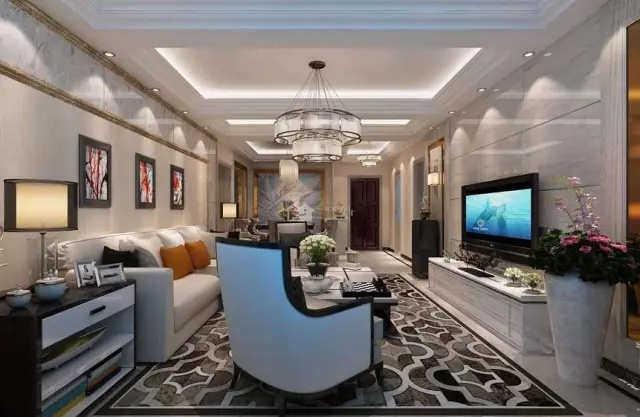去除霉味

家居装修中常会碰到乳胶漆因为干得慢、在潮热天气中会发霉变味的问题。对此，装修公司通用的办法是开空调抽湿，抽走空气中的水分；家具柜门不关 闭，保持通风。装修后，还会遇到各种气味散不

出去的问题，影响人们的健康。装修公司的建议是，装修后多摆绿色植物，或者在房间内放两三个柠檬、桔子、香 蕉，均可达到快速去味的效果。

应把暖气片考虑在内

这一点很多人容易忽视掉。夏季的炎热让大家很容易忽略掉这个问题，夏天不会用到暖气，但是在今后的居住中，您就会感觉到暖气的重要了。而且家庭装修工程有“不可重复性”，因此您无论何时装

修，都要把暖气的更换考虑在内。

结语：看完夏季装修的五大好处和注意事项，你终于知道为什么夏季也是装修的好时段了吧？赶快趁着夏天装修好，秋天能够通风透气，春节就能入住新家啦！

`声明：本文由入驻焦点开放平台的作者撰写，除焦点官方账号外，观点仅代表作者本人，不代表焦点立场错误信息举报电话： 400-099-0099，邮箱：jubao@vip.sohu.com，或点此进行意见反馈，或点此进行举报投诉。`A B C D E F G H J K L M N P Q R S T W X Y Z
A - B - C - D - E
• A
• 鞍山
• 安庆
• 安阳
• 安顺
• 安康
• 澳门
• B
• 北京
• 保定
• 包头
• 巴彦淖尔
• 本溪
• 蚌埠
• 亳州
• 滨州
• 北海
• 百色
• 巴中
• 毕节
• 保山
• 宝鸡
• 白银
• 巴州
• C
• 承德
• 沧州
• 长治
• 赤峰
• 朝阳
• 长春
• 常州
• 滁州
• 池州
• 长沙
• 常德
• 郴州
• 潮州
• 崇左
• 重庆
• 成都
• 楚雄
• 昌都
• 慈溪
• 常熟
• D
• 大同
• 大连
• 丹东
• 大庆
• 东营
• 德州
• 东莞
• 德阳
• 达州
• 大理
• 德宏
• 定西
• 儋州
• 东平
• E
• 鄂尔多斯
• 鄂州
• 恩施
F - G - H - I - J
• F
• 抚顺
• 阜新
• 阜阳
• 福州
• 抚州
• 佛山
• 防城港
• G
• 赣州
• 广州
• 桂林
• 贵港
• 广元
• 广安
• 贵阳
• 固原
• H
• 邯郸
• 衡水
• 呼和浩特
• 呼伦贝尔
• 葫芦岛
• 哈尔滨
• 黑河
• 淮安
• 杭州
• 湖州
• 合肥
• 淮南
• 淮北
• 黄山
• 菏泽
• 鹤壁
• 黄石
• 黄冈
• 衡阳
• 怀化
• 惠州
• 河源
• 贺州
• 河池
• 海口
• 红河
• 汉中
• 海东
• 怀来
• I
• J
• 晋中
• 锦州
• 吉林
• 鸡西
• 佳木斯
• 嘉兴
• 金华
• 景德镇
• 九江
• 吉安
• 济南
• 济宁
• 焦作
• 荆门
• 荆州
• 江门
• 揭阳
• 金昌
• 酒泉
• 嘉峪关
K - L - M - N - P
• K
• 开封
• 昆明
• 昆山
• L
• 廊坊
• 临汾
• 辽阳
• 连云港
• 丽水
• 六安
• 龙岩
• 莱芜
• 临沂
• 聊城
• 洛阳
• 漯河
• 娄底
• 柳州
• 来宾
• 泸州
• 乐山
• 六盘水
• 丽江
• 临沧
• 拉萨
• 林芝
• 兰州
• 陇南
• M
• 牡丹江
• 马鞍山
• 茂名
• 梅州
• 绵阳
• 眉山
• N
• 南京
• 南通
• 宁波
• 南平
• 宁德
• 南昌
• 南阳
• 南宁
• 内江
• 南充
• P
• 盘锦
• 莆田
• 平顶山
• 濮阳
• 攀枝花
• 普洱
• 平凉
Q - R - S - T - W
• Q
• 秦皇岛
• 齐齐哈尔
• 衢州
• 泉州
• 青岛
• 清远
• 钦州
• 黔南
• 曲靖
• 庆阳
• R
• 日照
• 日喀则
• S
• 石家庄
• 沈阳
• 双鸭山
• 绥化
• 上海
• 苏州
• 宿迁
• 绍兴
• 宿州
• 三明
• 上饶
• 三门峡
• 商丘
• 十堰
• 随州
• 邵阳
• 韶关
• 深圳
• 汕头
• 汕尾
• 三亚
• 三沙
• 遂宁
• 山南
• 商洛
• 石嘴山
• T
• 天津
• 唐山
• 太原
• 通辽
• 铁岭
• 泰州
• 台州
• 铜陵
• 泰安
• 铜仁
• 铜川
• 天水
• 天门
• W
• 乌海
• 乌兰察布
• 无锡
• 温州
• 芜湖
• 潍坊
• 威海
• 武汉
• 梧州
• 渭南
• 武威
• 吴忠
• 乌鲁木齐
X - Y - Z
• X
• 邢台
• 徐州
• 宣城
• 厦门
• 新乡
• 许昌
• 信阳
• 襄阳
• 孝感
• 咸宁
• 湘潭
• 湘西
• 西双版纳
• 西安
• 咸阳
• 西宁
• 仙桃
• 西昌
• Y
• 运城
• 营口
• 盐城
• 扬州
• 鹰潭
• 宜春
• 烟台
• 宜昌
• 岳阳
• 益阳
• 永州
• 阳江
• 云浮
• 玉林
• 宜宾
• 雅安
• 玉溪
• 延安
• 榆林
• 银川
• Z
• 张家口
• 镇江
• 舟山
• 漳州
• 淄博
• 枣庄
• 郑州
• 周口
• 驻马店
• 株洲
• 张家界
• 珠海
• 湛江
• 肇庆
• 中山
• 自贡
• 资阳
• 遵义
• 昭通
• 张掖
• 中卫

1室1厅1厨1卫1阳台

1
2
3
4
5

0
1
2

1

1

0
1
2
3报名成功，资料已提交审核A B C D E F G H J K L M N P Q R S T W X Y Z
A - B - C - D - E
• A
• 鞍山
• 安庆
• 安阳
• 安顺
• 安康
• 澳门
• B
• 北京
• 保定
• 包头
• 巴彦淖尔
• 本溪
• 蚌埠
• 亳州
• 滨州
• 北海
• 百色
• 巴中
• 毕节
• 保山
• 宝鸡
• 白银
• 巴州
• C
• 承德
• 沧州
• 长治
• 赤峰
• 朝阳
• 长春
• 常州
• 滁州
• 池州
• 长沙
• 常德
• 郴州
• 潮州
• 崇左
• 重庆
• 成都
• 楚雄
• 昌都
• 慈溪
• 常熟
• D
• 大同
• 大连
• 丹东
• 大庆
• 东营
• 德州
• 东莞
• 德阳
• 达州
• 大理
• 德宏
• 定西
• 儋州
• 东平
• E
• 鄂尔多斯
• 鄂州
• 恩施
F - G - H - I - J
• F
• 抚顺
• 阜新
• 阜阳
• 福州
• 抚州
• 佛山
• 防城港
• G
• 赣州
• 广州
• 桂林
• 贵港
• 广元
• 广安
• 贵阳
• 固原
• H
• 邯郸
• 衡水
• 呼和浩特
• 呼伦贝尔
• 葫芦岛
• 哈尔滨
• 黑河
• 淮安
• 杭州
• 湖州
• 合肥
• 淮南
• 淮北
• 黄山
• 菏泽
• 鹤壁
• 黄石
• 黄冈
• 衡阳
• 怀化
• 惠州
• 河源
• 贺州
• 河池
• 海口
• 红河
• 汉中
• 海东
• 怀来
• I
• J
• 晋中
• 锦州
• 吉林
• 鸡西
• 佳木斯
• 嘉兴
• 金华
• 景德镇
• 九江
• 吉安
• 济南
• 济宁
• 焦作
• 荆门
• 荆州
• 江门
• 揭阳
• 金昌
• 酒泉
• 嘉峪关
K - L - M - N - P
• K
• 开封
• 昆明
• 昆山
• L
• 廊坊
• 临汾
• 辽阳
• 连云港
• 丽水
• 六安
• 龙岩
• 莱芜
• 临沂
• 聊城
• 洛阳
• 漯河
• 娄底
• 柳州
• 来宾
• 泸州
• 乐山
• 六盘水
• 丽江
• 临沧
• 拉萨
• 林芝
• 兰州
• 陇南
• M
• 牡丹江
• 马鞍山
• 茂名
• 梅州
• 绵阳
• 眉山
• N
• 南京
• 南通
• 宁波
• 南平
• 宁德
• 南昌
• 南阳
• 南宁
• 内江
• 南充
• P
• 盘锦
• 莆田
• 平顶山
• 濮阳
• 攀枝花
• 普洱
• 平凉
Q - R - S - T - W
• Q
• 秦皇岛
• 齐齐哈尔
• 衢州
• 泉州
• 青岛
• 清远
• 钦州
• 黔南
• 曲靖
• 庆阳
• R
• 日照
• 日喀则
• S
• 石家庄
• 沈阳
• 双鸭山
• 绥化
• 上海
• 苏州
• 宿迁
• 绍兴
• 宿州
• 三明
• 上饶
• 三门峡
• 商丘
• 十堰
• 随州
• 邵阳
• 韶关
• 深圳
• 汕头
• 汕尾
• 三亚
• 三沙
• 遂宁
• 山南
• 商洛
• 石嘴山
• T
• 天津
• 唐山
• 太原
• 通辽
• 铁岭
• 泰州
• 台州
• 铜陵
• 泰安
• 铜仁
• 铜川
• 天水
• 天门
• W
• 乌海
• 乌兰察布
• 无锡
• 温州
• 芜湖
• 潍坊
• 威海
• 武汉
• 梧州
• 渭南
• 武威
• 吴忠
• 乌鲁木齐
X - Y - Z
• X
• 邢台
• 徐州
• 宣城
• 厦门
• 新乡
• 许昌
• 信阳
• 襄阳
• 孝感
• 咸宁
• 湘潭
• 湘西
• 西双版纳
• 西安
• 咸阳
• 西宁
• 仙桃
• 西昌
• Y
• 运城
• 营口
• 盐城
• 扬州
• 鹰潭
• 宜春
• 烟台
• 宜昌
• 岳阳
• 益阳
• 永州
• 阳江
• 云浮
• 玉林
• 宜宾
• 雅安
• 玉溪
• 延安
• 榆林
• 银川
• Z
• 张家口
• 镇江
• 舟山
• 漳州
• 淄博
• 枣庄
• 郑州
• 周口
• 驻马店
• 株洲
• 张家界
• 珠海
• 湛江
• 肇庆
• 中山
• 自贡
• 资阳
• 遵义
• 昭通
• 张掖
• 中卫• 手机• 分享
• 设计
免费设计
• 计算器
装修计算器
• 入驻
合作入驻
• 联系
联系我们
• 置顶
返回顶部